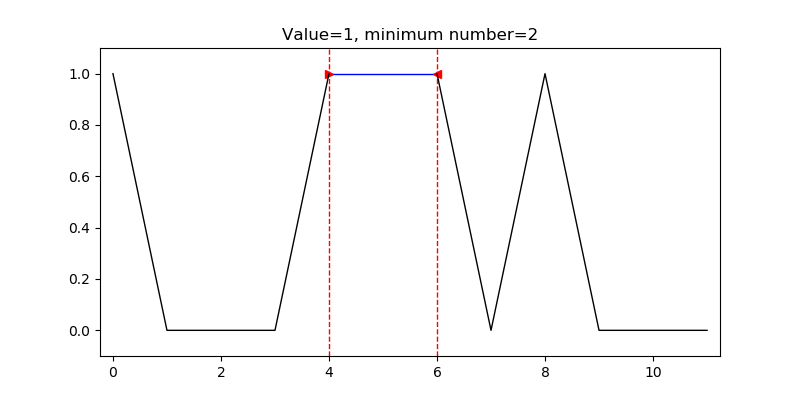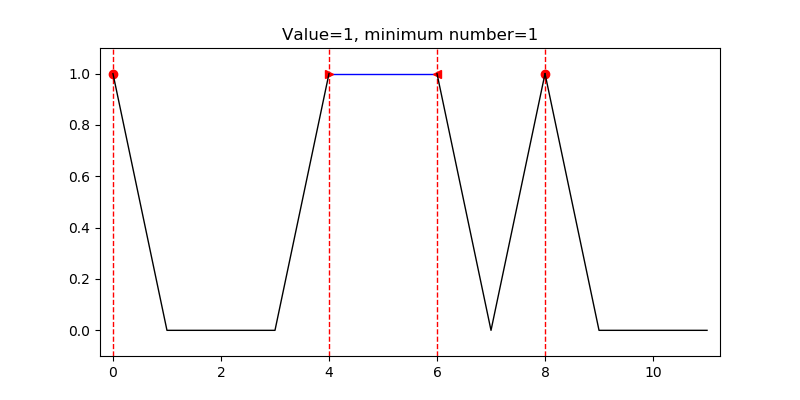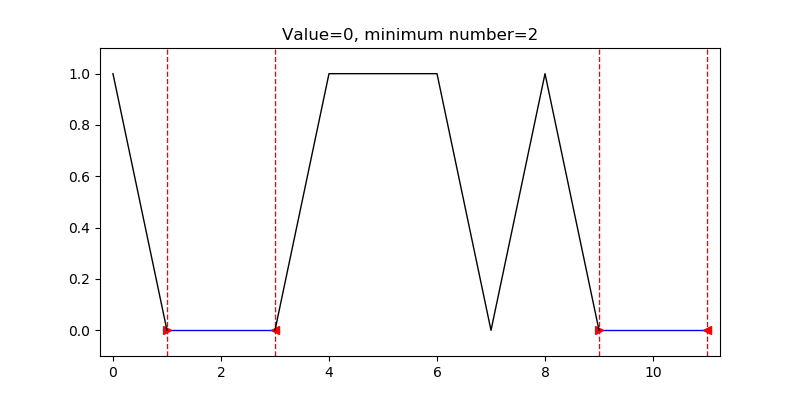# Replace missing data in different ways¶

Marcos Duarte
Laboratory of Biomechanics and Motor Control (http://demotu.org/)
Federal University of ABC, Brazil

In :
import numpy as np
%matplotlib notebook
import sys, os
sys.path.insert(1, r'./../functions')
from rep_missing import rep_missing


The function rep_missing.py replaces missing data in different ways.

The signature of rep_missing.py is:

y = rep_missing(x, value=np.nan, new_value='interp', max_alert=100)

In :
help(rep_missing)

Help on function rep_missing in module rep_missing:

Replace missing data in different ways.

Parameters
----------
x : 1D numpy array_like
data
value : number, optional. Default = np.nan
Value to be found in x marking missing data
new_value : number or string, optional. Default = 'interp'
Value or string for the method to use for replacing missing data:
'delete': delete missing data
new_value: replace missing data with new_value
'mean': replace missing data with the mean of the rest of the data
'median': replace missing data with the median of the rest of the data
'interp': replace missing data by linear interpolating over them.
max_alert : number, optional. Default = 100
Minimal number of sequential data for a message to be printed with
information about the continuous missing data.
Set to 0 to not print any message.

Returns
-------
y : 1D numpy array_like
1D array similar to x but with missing data replaced according
to value or method in new_value.

References
----------
..  http://nbviewer.jupyter.org/github/demotu/BMC/blob/master/notebooks/rep_missing.ipynb

Examples
--------
>>> x = [1, 0, 0, 0, 1, 1, 1, 0, 1, 0, 0, 0]


In :
x = [1, 2, np.nan, 4, 20, np.nan, np.nan, 0, 1]

In :
rep_missing(x, 0, new_value='mean')

Out:
array([ 1. ,  2. ,  nan,  4. , 20. ,  nan,  nan,  5.6,  1. ])
In :
np.s

Out:
float
In :
detect_seq(x, 0, index=True, min_seq=2)

Out:
array([[ 1,  3],
[ 9, 11]])
In :
detect_seq(x, 10)

Out:
array([False, False, False, False, False, False, False, False, False,
False, False, False])
In :
detect_seq(x, 10, index=True)

Out:
array([], shape=(0, 2), dtype=int64)
In :
detect_seq(x, 1, index=True, min_seq=2, show=True)Out:
array([[4, 6]])
In :
detect_seq(x, 1, index=False, min_seq=1, show=True)Out:
array([ True, False, False, False,  True,  True,  True, False,  True,
False, False, False])
In :
detect_seq(x, 0, index=True, min_seq=2, show=True)Out:
array([[ 1,  3],
[ 9, 11]])
In :
detect_seq(x, 0, index=True, max_alert=2)

Sequential data equal or longer than 2: (, [1 3])
Sequential data equal or longer than 2: (, [ 9 11])

Out:
array([[ 1,  3],
[ 7,  7],
[ 9, 11]])

## Function rep_missing.py¶

In [ ]:
# %load ./../functions/rep_missing.py
#!/usr/bin/env python

"""Replace missing data in different ways."""

import numpy as np
from detect_seq import detect_seq
try:
from tnorm import tnorm
except:

__author__ = 'Marcos Duarte, https://github.com/demotu/BMC'
__version__ = 'rep_missing.py v.1.0.0 2019/03/17'

"""Replace missing data in different ways.

Parameters
----------
x : 1D numpy array_like
data
value : number, optional. Default = np.nan
Value to be found in x marking missing data
new_value : number or string, optional. Default = 'interp'
Value or string for the method to use for replacing missing data:
'delete': delete missing data
new_value: replace missing data with new_value
'mean': replace missing data with the mean of the rest of the data
'median': replace missing data with the median of the rest of the data
'interp': replace missing data by linear interpolating over them.
max_alert : number, optional. Default = 100
Minimal number of sequential data for a message to be printed with
information about the continuous missing data.
Set to 0 to not print any message.

Returns
-------
y : 1D numpy array_like
1D array similar to x but with missing data replaced according
to value or method in new_value.

References
----------
..  http://nbviewer.jupyter.org/github/demotu/BMC/blob/master/notebooks/rep_missing.ipynb

Examples
--------
>>> x = [1, 0, 0, 0, 1, 1, 1, 0, 1, 0, 0, 0]

"""

y = np.asarray(x)

if idx.any():
if new_value == 'delete':
y = y[~idx]
elif np.isreal(new_value) or np.iscomplex(new_value):
y[idx] = new_value
elif new_value == 'mean':
y[idx] = np.nanmean(y)
elif new_value == 'median':
y[idx] = np.nanmedian(y)
elif new_value == 'interp':
y[idx] = np.nan
y, t, indie = tnorm(y, step=0, k=1, smooth=0, nan_at_ext='replace')

return y

In [ ]: